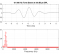## z-transform: Confused on how to find ROC of H(z)Started by 5 years ago4 replieslatest reply 5 years ago2016 views

Hi, I've been studying the z-transform for my DSP course and I know that the ROC of Y(z) is simply the intersection of X(z) and H(z). Furthermore, I know that Y(z) can be found via the convolution property: Y(z) = X(z)*H(z), where * denotes simple multiplication.

My confusion is in finding the ROC of H(z); I know that H(z) = Y(z)/X(z) via the same convolution property, but how would I determine its ROC assuming that the ROC of X(z) and the ROC of Y(z) are known? Also, How would I know if pole-zero cancellation occurs, thus affecting the ROC?

Thank you very much, your help is greatly appreciated.

[ - ]I never got deeply into that ROC stuff, because I've never been able to extract much physical meaning from it.

In general, I'm not sure that you can determine the ROC of $$H(z)$$ from those of $$X(z)$$ and $$Y(z)$$.

So much for your main question.  Sorry.

For your other question, about pole-zero cancellation: you can't, unless someone invents some way of decorating a transfer function.  Proper transfer functions lose information about pole-zero cancellations.  You're simply out of luck on that one.  This is part of why people invented state-space representations for systems: so they could see that systems had uncontrollable or unobservable modes, and therefore pole-zero cancellations.

[ - ]Hello, Mr. Westcott:

Your comments are extremely interesting?  Could you please elaborate on them?  In particular the ideas of "decorating a transfer function" and how proper transfer functions lose information about pole-zero cancellation.

Thank you.

[ - ]Hi, This is a great theoretical question. The ROC concept is applied to H(z) when performing the z-transform of the sequence h(n). Essentially the ROC defines the conditions for convergence of h(n), the impulse response of the system. The ROC does not involve X(z), the input, or Y(z), the output. When you write H(z) as Y(z)/X(z) you assume a steady state response which requires a stable H(z) which means the ROC has been already satisfied.

But if you want to know the ROC for an H(z) you have to find the poles, the roots, of the denominator polynomial in z.

BTW given we tend to design digital filters and the like with software, Matlab, etc., one doesn't tend to think about stability and ROC. Back in the day we had to manually plot poles and zeros of H(z) functions to make sure they were stable. But now the software does it for you.

I hope this helps.

Regards

[ - ]